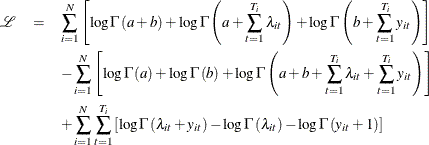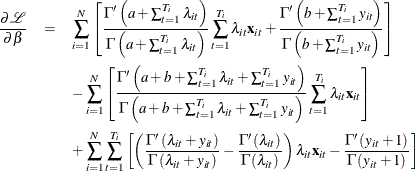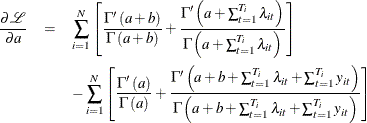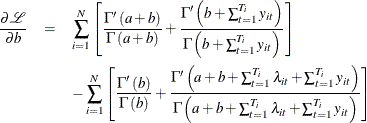The COUNTREG Procedure

Panel Data Analysis

Subsections:

Panel Data Poisson Regression with Fixed Effects

The count regression model for panel data can be derived from the Poisson regression model. Consider the multiplicative one-way panel data model,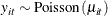where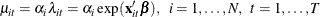Here,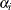are the individual effects.

In the fixed-effects model, theare unknown parameters. The fixed-effects model can be estimated by eliminatingby conditioning on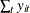.

In the random-effects model, theare independent and identically distributed (iid) random variables, in contrast to the fixed effects model. The random-effects model can then be estimated by assuming a distribution for.

In the Poisson fixed-effects model, conditional on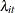and parameter,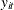is iid Poisson-distributed with parameter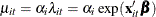, and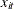does not include an intercept. Then, the conditional joint density for the outcomes within the ith panel is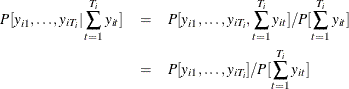Becauseis iid Poisson(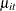),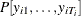is the product of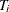Poisson densities. Also,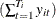is Poisson(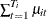). Then,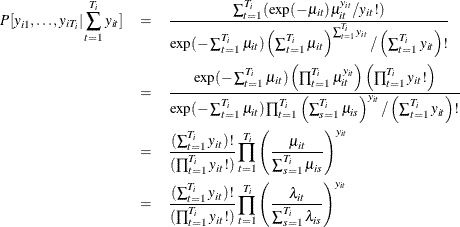Thus, the conditional log-likelihood function of the fixed-effects Poisson model is given by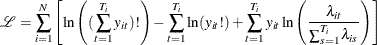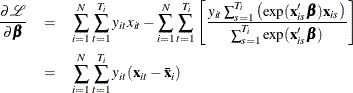where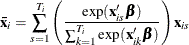Panel Data Poisson Regression with Random Effects

In the Poisson random-effects model, conditional onand parameter,is iid Poisson-distributed with parameter, and the individual effects,, are assumed to be iid random variables. The joint density for observations in all time periods for the ith individual,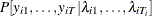, can be obtained after the density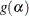of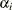is specified.

Let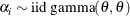so that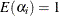and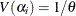: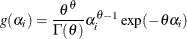Let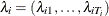. Becauseis conditional onand parameteris iid Poisson(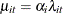), the conditional joint probability for observations in all time periods for the ith individual,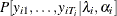, is the product ofPoisson densities: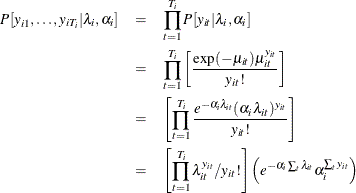Then, the joint density for the ith panel conditional on just thecan be obtained by integrating out: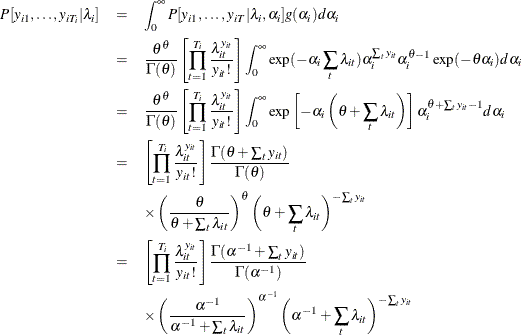where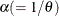is the overdispersion parameter. This is the density of the Poisson random-effects model with gamma-distributed random effects. For this distribution,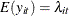and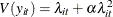; that is, there is overdispersion.

Then the log-likelihood function is written as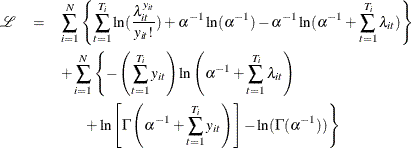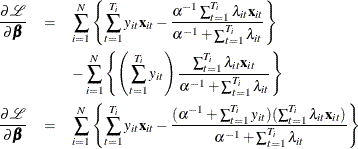and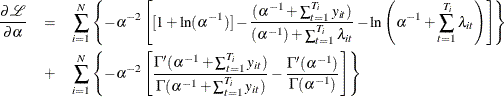where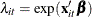,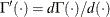andis the digamma function.

Panel Data Negative Binomial Regression with Fixed Effects

This section shows the derivation of a negative binomial model with fixed effects. Keep the assumptions of the Poisson-distributed dependent variable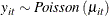But now let the Poisson parameter be random with gamma distribution and parameters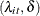,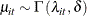where one of the parameters is the exponentially affine function of independent variables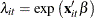. Use integration by parts to obtain the distribution of,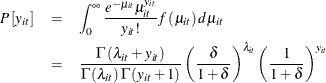which is a negative binomial distribution with parameters. Conditional joint distribution is given as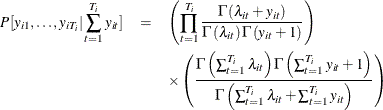Hence, the conditional fixed-effects negative binomial log-likelihood is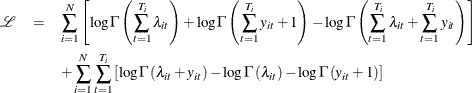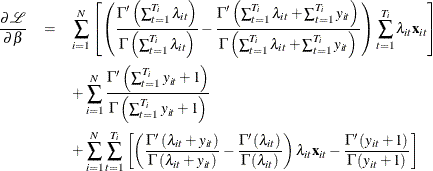Panel Data Negative Binomial Regression with Random Effects

This section describes the derivation of negative binomial model with random effects. Supposewith the Poisson parameter distributed as gamma,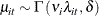where its parameters are also random: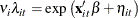Assume that the distribution of a function of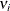is beta with parameters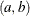: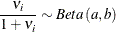Explicitly, the beta density with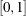domain is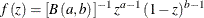where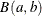is the beta function. Then, conditional joint distribution of dependent variables is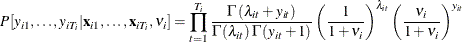Integrating out the variableyields the following conditional distribution function: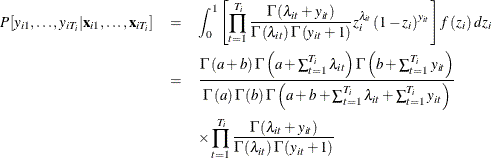Consequently, the conditional log-likelihood function for a negative binomial model with random effects is